##### Differential Equations For Dummies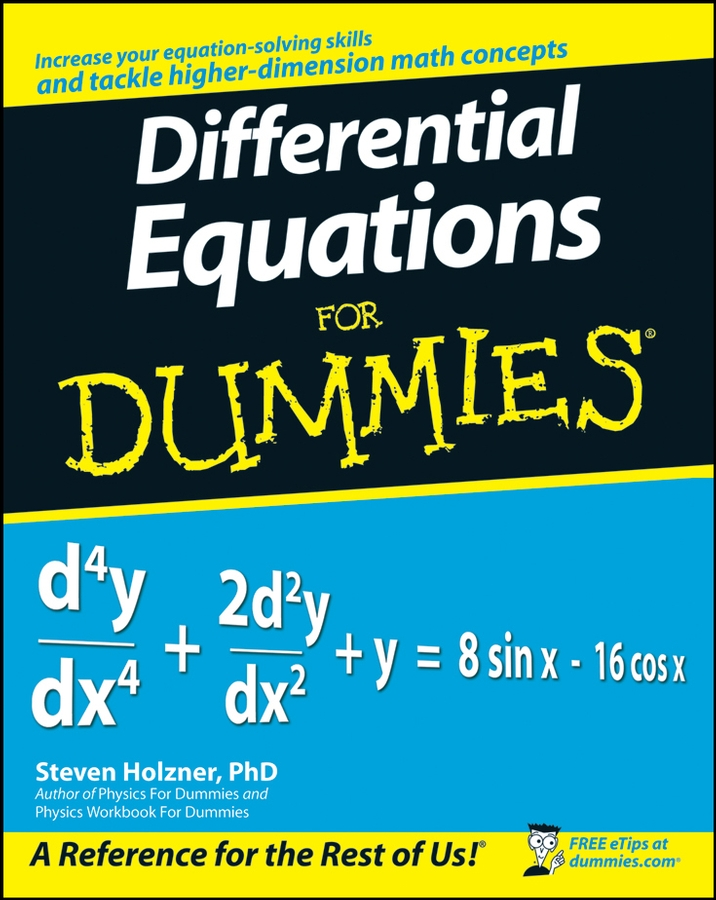An ellipse is a set of points on a plane, creating an oval, curved shape, such that the sum of the distances from any point on the curve to two fixed points (the foci) is a constant (always the same). An ellipse is basically a circle that has been squished either horizontally or vertically.

Graphically speaking, you must know two different types of ellipses: horizontal and vertical. A horizontal ellipse is short and fat; a vertical one is tall and skinny. Each type of ellipse has these main parts:

• The point in the middle of the ellipse is called the center and is named (h, v) just like the vertex of a parabola and the center of a circle.

• The major axis is the line that runs through the center of the ellipse the long way. The variable a is the letter used to name the distance from the center to the ellipse on the major axis. The endpoints of the major axis are on the ellipse and are called vertices.

• The minor axis is perpendicular to the major axis and runs through the center the short way. The variable b is the letter used to name the distance to the ellipse from the center on the minor axis. Because the major axis is always longer than the minor one, a > b. The endpoints on the minor axis are called co-vertices.

• The foci are the two points that dictate how fat or how skinny the ellipse is. They are always located on the major axis, and can be found by the following equation: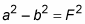where a and b are mentioned as in the preceding bullets, and F is the distance from the center to each focus.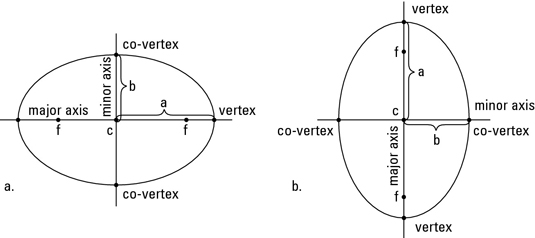This figure shows a horizontal ellipse and a vertical ellipse with their parts labeled. Notice that the length of the major axis is 2a, and the length of the minor axis is 2b. This figure also shows the correct placement of the foci — always on the major axis.

Two types of equations apply to ellipses, depending on whether they’re horizontal or vertical:

The horizontal equation is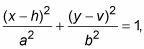with the center at (h, v), major axis of 2a, and minor axis of 2b.

The vertical equation is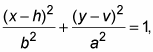with the same parts — although a and b have switched places.

When the bigger number a is under x, the ellipse is horizontal; when the bigger number is under y, it’s vertical.

You have to be prepared to not only graph ellipses, but also to name all their parts. If a problem asks you to calculate the parts of an ellipse, you have to be ready to deal with some ugly square roots and/or decimals. The following presents the parts for both horizontal and vertical ellipses.

Horizontal Ellipse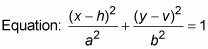Center: (h, v)

Vertices: (h ± a, v)

Co-vertices: (h, v ± b)

Length of Major Axis: 2a

Length of Minor Axis: 2b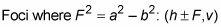Vertical Ellipse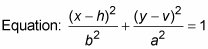Center: (h, v)

Vertices: (h, v ± a)

Co-vertices: (h ± b, v)

Length of Major Axis: 2a

Length of Minor Axis: 2b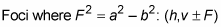To find the vertices in a horizontal ellipse, use (h ± a, v); to find the co-vertices, use (h, v ± b). A vertical ellipse has vertices at (h, v ± a) and co-vertices at (h ± b, v).

For example, look at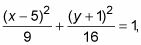which is already in the proper form to graph. You know that h = 5 and v = –1 (switching the signs inside the parentheses).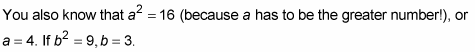This example is a vertical ellipse because the bigger number is under y, so be sure to use the correct formula. This equation has vertices at (5, –1 ± 4), or (5, 3) and (5, –5). It has co-vertices at (5 ± 3, –1), or (8, –1) and (2, –1).

The major axis in a horizontal ellipse is given by the equation y = v; the minor axis is given by x = h. The major axis in a vertical ellipse is represented by x = h; the minor axis is represented by y = v. The length of the major axis is 2a, and the length of the minor axis is 2b.

You can calculate the distance from the center to the foci in an ellipse (either variety) by using the equation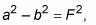where F is the distance from the center to each focus. The foci always appear on the major axis at the given distance (F) from the center.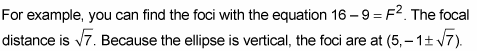What if the elliptical equation you’re given isn’t in standard form? Take a look at the example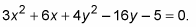Follow these steps to put the equation in standard form:

1. Add the constant to the other side.

This gives you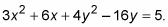2. Complete the square.

You need to factor out two different constants now — the different coefficients for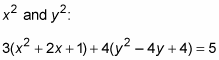3. Balance the equation by adding the new terms to the other side.

In other words,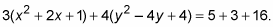Note: Adding 1 and 4 inside the parentheses really means adding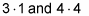to each side, because you must multiply by the coefficient before adding it to the right side.

4. Factor the left side of the equation and simplify right.

You now have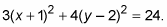5. Divide the equation by the constant on the right to get 1 and then reduce the fractions.

You now have the form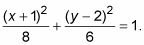6. Determine if the ellipse is horizontal or vertical.

Because the bigger number is under x, this ellipse is horizontal.

7. Find the center and the length of the major and minor axes.

The center is located at (h, v), or (–1, 2).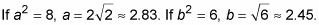8. Graph the ellipse to determine the vertices and co-vertices.

Go to the center first and mark the point.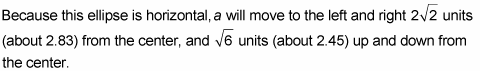Plotting these points will locate the vertices of the ellipse.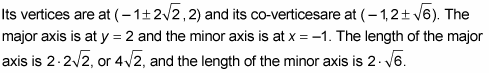9. Plot the foci of the ellipse.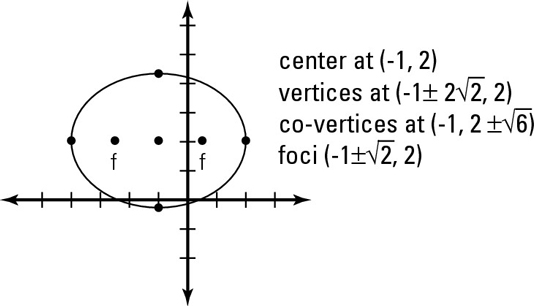You determine the focal distance from the center to the foci in this ellipse with the equation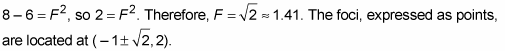The above figure shows all the parts of this ellipse in its fat glory.